Next: N narrow slits Up: Two narrow slits Previous: Analysis

### Interpretation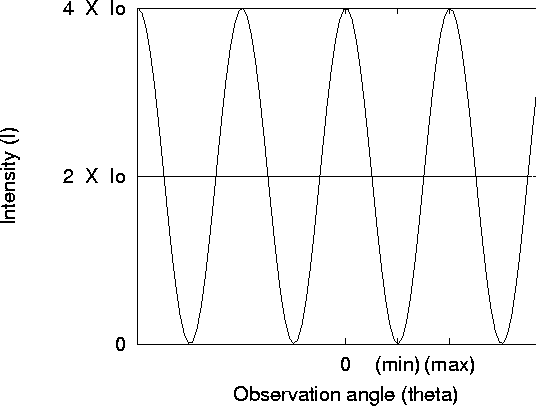Figure 5: Intensity as a function of observation angle for a double-slit experiment

Eqs. 13 & 14 give the intensity as a function of angle of observation. Figure 5 shows the resulting pattern. Three aspects of the result are noteworthy.

• The peak intensity,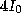, is greater than the intensity we expect from simply summing the intensity of two slits. However, there are also places where the intensity drops to zero. In fact, on average, the intensity is exactly what we would expect from two slits,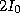.
• The maxima in the pattern occur when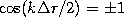, which means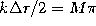, where M is some integer. This condition becomes then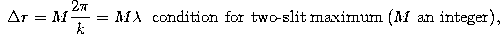which means simply that the path difference is an even number of wavelengths so that the two waves arriving at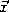are in phase, adding together constructively. Under these conditions, the amplitude will then be exactly double, and because the intensity goes like the square of the amplitude, we expect 4 times the intensity, as Eq. 13 predicts.

• The minima (where there is zero intensity, actually) occur when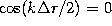, which means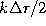is an odd half multiple of,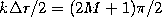, where M is any integer. This conditions then becomes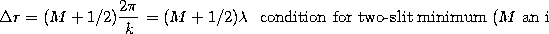which simply means that the path difference is off by exactly one-half of a wavelength, so that the two waves arrive exactly out of phase, and thus cancel completely, leaving zero amplitude and zero intensity.

Tomas Arias
Thu Sep 13 15:26:14 EDT 2001